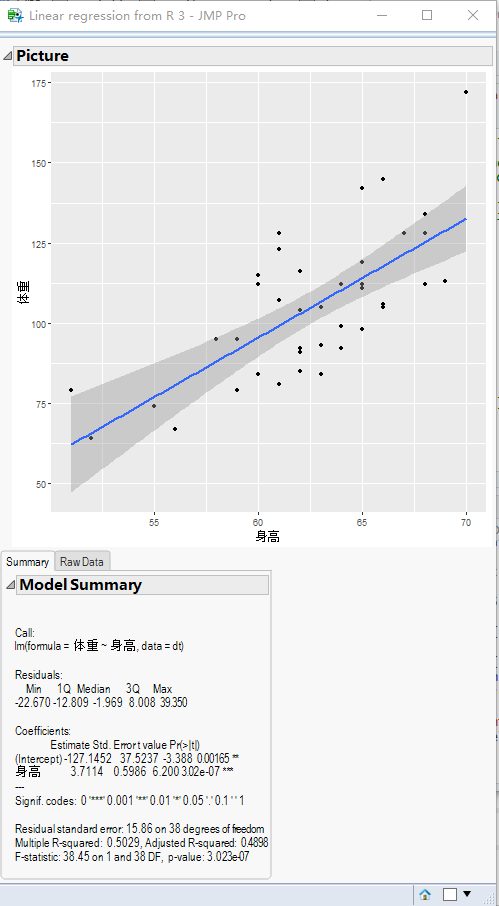Memu refer below

# Interactive: JMP and R

### General Summary

There’s many statistical analysis software, jmp and R are two of them.

### R input JMP file

Also, there’s many ways to import data from other format to R, but there’s no directly way to import jmp data into R.

library(RDCOMClient)

jmp <- COMCreate(“JMP.Application”)

doc = jmp$OpenDocument(“C:/Demo.jmp”) doc$SaveAs(“d:/Demo.csv”)

file.remove(“d:/Demo.csv”)

### JMP call R

JMP can also call R and return the calculated result or graphics to JMP.

Set Environment Variable( “R_HOME”, “E:\Program Files\R” );

dt = open(“\$SAMPLE_DATA/Big Class.jmp”); // Open a dataset

R init(); // Initialize a R session R Send(dt); // Send the dataset to R Close(dt); // Close the data table of JMP

R_stdout = Log Capture(R Submit(” summary(lm(体重~身高,data=dt)) “));

R Submit(”

library(ggplot2)

ggplot(dt,aes(x=身高,y=体重))+geom_point()+geom_smooth(method=lm)

”); // Execute R code to plot a scatter plot with linear line plot = R Get Graphics(“png”); // Get the graphics object